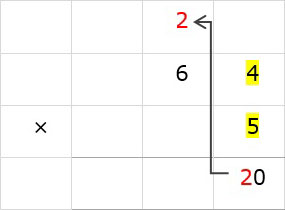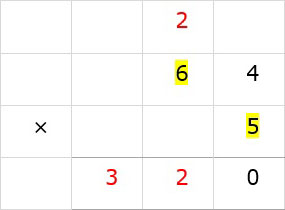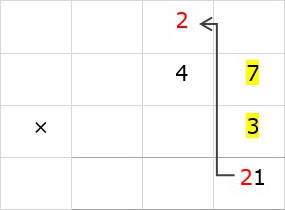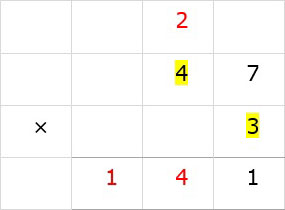# Multiplication with carry

#### Complete Python Prime Pack

9 Courses     2 eBooks

#### Artificial Intelligence & Machine Learning Prime Pack

6 Courses     1 eBooks

#### Java Prime Pack

9 Courses     2 eBooks

Multiply 64 × 5

### Solution

Step 1:

First we multiply the ones digit of 64 with 5, i.e., 5 × 4 = 20. We write down 0 below ones column and carry over 2 to tens column.Step 2:

Then we multiply the tens digit of 64 with 5, i.e., 5 × 6 = 30

In tens column 30 + 2 = 32. We write 2 below tens column and 3 below hundreds column as shown.So 64 × 5 = 320

Multiply 47 × 3

### Solution

Step 1:

First we multiply 7 of 47 with 3; 3 × 7 = 21. We write 1 below ones column and carry 2 to tens column.Step 2:

Then we multiply 4 of 47 with 3; 3 × 4 = 12

12 + 2 = 14. We write 4 below tens column and 1 in hundreds column.So 47 × 3 = 141